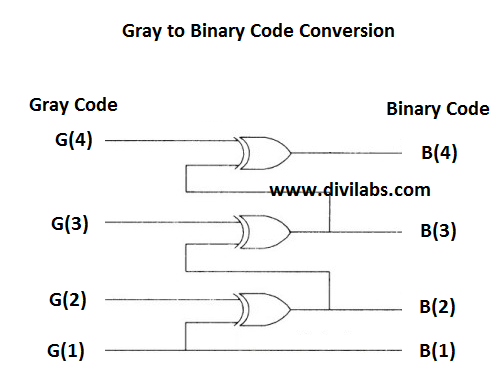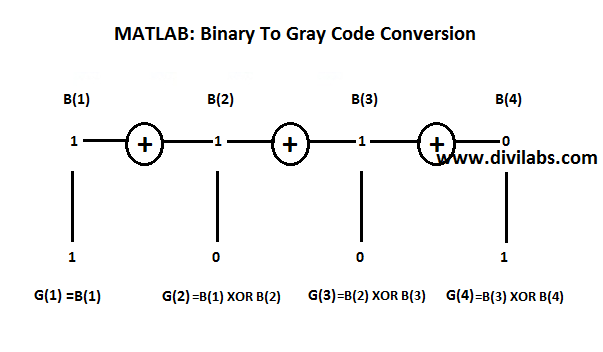# Gray To Binary Code Conversion in MATLAB without using MATLAB's Inbuilt Function

In the previous post, Binary To Gray Code Conversion in MATLAB without using MATLAB's Inbuilt Function, we have converted Binary code to Gray code.
Now its time for performing the reverse process.
The process of converting a Gray code to a Binary code also requiring XORing, but with the resultant bits with the input bits, rather than a pair or input bits that we have done previously while converting a Binary code to Gray one.

This process can be illustrated as:GATE Level Logic Diagram of Gray to Binary Code ConversionMATLAB: How the Implementation of Gray Code To Binary Code conversion is done
Suppose we had originally a binary bit stream of 30 bits,
a =
0     1     0     0     1     1     1     0     1     1     1     0     1     1     0     0     1     1     0     1     1     0     0     0     0     0     0     1     0     1

After Gray Coding the Bit Stream we got like this,
g =
0     1     1     0     1     0     0     1     1     0     0     1     1     0     1     0     1     0     1     1     0     1     0     0     0     0     0     1     1     1

So to check our code is working well or not we will give 'g' as an input to recover the original binary bit sequence 'a'. You can find the opposite here, Binary To Gray Code Conversion in MATLAB without using MATLAB's Inbuilt Function.

MATLAB code for Conversion of Gray Code to Binary Code is:
%if you want to use your own sequence just declare or gather the variable 'g' before the start of following lines
x(:,1) = g(:,1); %copying the 1st gray bit as it is, to the resultant vector

for i = 2:size(g,2),
x(:,i) = xor( x(:,i-1), g(:,i) );
%XORing the following bits as per the procedure
end

The resultant value of x is,
x =
0     1     0     0     1     1     1     0     1     1     1     0     1     1     0     0     1     1     0     1     1     0     0     0     0     0     0     1     0     1

On comparing with the original Binary Sequence 'a' we find that 'x' is same to it. So our code is converting the gray code to binary code very well.

# Binary To Gray Code Conversion in MATLAB without using MATLAB's Inbuilt Function

How a Binary code (of any length) is converted to a Gray Code, its a simple process requiring XORing  of 2 bits & saving the resultant bit in a new place. I have tried to explain this process by the following 2 diagrams. In this I have taken & assumed 4 bit arbitrary binary code, and operating it with XOR gates to convert to Gray code.
B(1), B(2), B(3) & B(4) collectively denotes the 4 bit binary, of which B(1) is the MSB. G(1), G(2), G(3) & G(4) collectively denotes the 4 bit gray code.GATE Level Logic Diagram of Binary to Gray Code ConversionMATLAB: How the Implementation of Binary Code To Gray Code conversion is done

The MATLAB code below for conversion of Binary Code to Gray code, will work for any binary vector or matrix, i.e., you not need to worry about the conversion of 'n x m' binary matrix to a 'n x 1' binary vector first, you simply feed in & the code will give the resultant Gray Code Matrix for the same Binary Code Matrix. The code below will then process row wise in order to calculate the Gray Code.

Suppose we are having a binary 10 x 10 matrix, so the code below will take 1 binary value row at 1 time then process it to give a equal sized gray coded row. The similar thing will be repeated with each row of that matrix. Even if its a single vector of 'n x 1' size this code will work.

MATLAB Code for conversion of Binary Code to Gray Code:
a=rand(10,10)>0.5;
%the variable 'a' contains the binary values in terms of a matrix currently its a 100x100 binary matrix %you can provide your own matrix of any size but remember it will calculate the gray code row wise
g(:,1) = a(:,1); %assign the 1st element of each row to the resultant 'g', as it is.
for i = 2:size(a,2)
g(:,i) = xor( a(:,i-1), a(:,i) );%processing as per the procedure
end

Example Execution:
With a 10x10 binary matrix,
a = %the binary matrix
0     1     1     0     0     1     1     1     1     1
0     0     0     0     1     0     0     1     1     0
0     0     0     1     1     0     0     1     1     1
1     0     0     1     0     1     1     1     1     1
1     1     1     0     0     0     0     0     1     1
1     0     0     0     0     1     0     1     0     1
0     1     1     0     0     1     0     0     0     1
1     0     1     0     0     1     0     1     0     1
1     0     1     1     1     1     0     1     1     0
1     0     1     0     1     0     0     0     0     1

g = %the gray coded matrix coded row wise
0     1     0     1     0     1     0     0     0     0
0     0     0     0     1     1     0     1     0     1
0     0     0     1     0     1     0     1     0     0
1     1     0     1     1     1     0     0     0     0
1     0     0     1     0     0     0     0     1     0
1     1     0     0     0     1     1     1     1     1
0     1     0     1     0     1     1     0     0     1
1     1     1     1     0     1     1     1     1     1
1     1     1     0     0     0     1     1     0     1
1     1     1     1     1     1     0     0     0     1

With a 1x30 binary vector,
a = %the binary vector
0     1     0     0     1     1     1     0     1     1     1     0     1     1     0     0     1     1     0     1     1     0     0     0     0     0     0     1     0     1

g = %the gray coded vector
0     1     1     0     1     0     0     1     1     0     0     1     1     0     1     0     1     0     1     1     0     1     0     0     0     0     0     1     1     1

Also Visit:
Gray To Binary Code Conversion in MATLAB without using MATLAB's Inbuilt Function